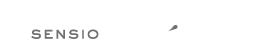# Rule `strict_param`¶

Functions should be used with `\$strict` param set to `true`.

## Description¶

The functions “array_keys”, “array_search”, “base64_decode”, “in_array” and “mb_detect_encoding” should be used with \$strict param.

## Warning¶

### Using this rule is risky¶

Risky when the fixed function is overridden or if the code relies on non-strict usage.

## Examples¶

### Example #1¶

```--- Original
+++ New
<?php
\$a = array_keys(\$b);
-\$a = array_search(\$b, \$c);
-\$a = base64_decode(\$b);
-\$a = in_array(\$b, \$c);
-\$a = mb_detect_encoding(\$b, \$c);
+\$a = array_search(\$b, \$c, true);
+\$a = base64_decode(\$b, true);
+\$a = in_array(\$b, \$c, true);
+\$a = mb_detect_encoding(\$b, \$c, true);
```

## Rule sets¶

The rule is part of the following rule set:

The logo is © 2010-2022 Sensio Labs